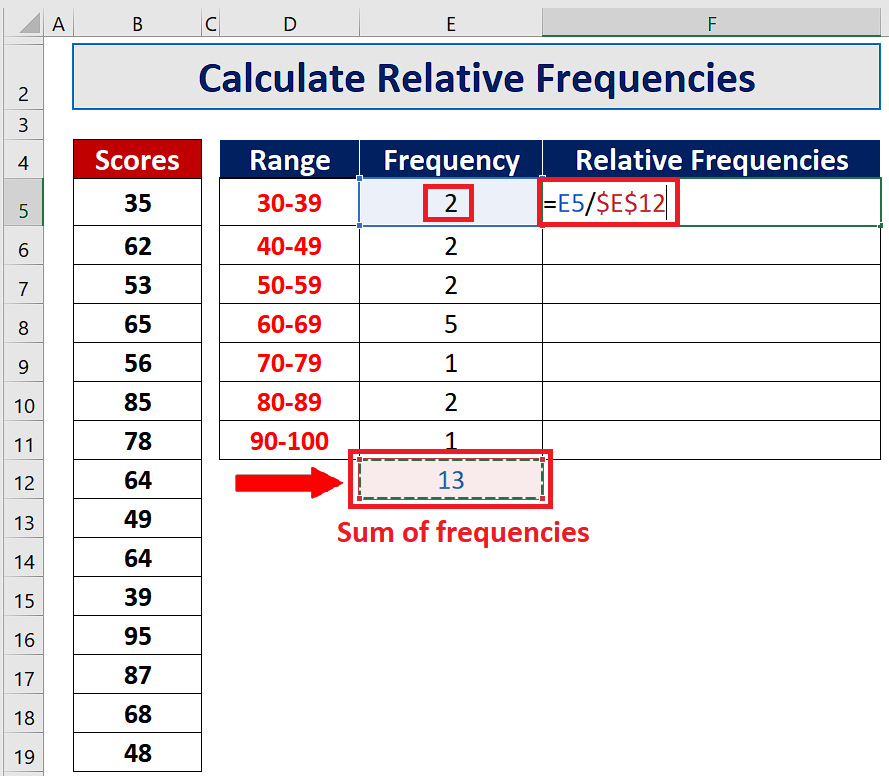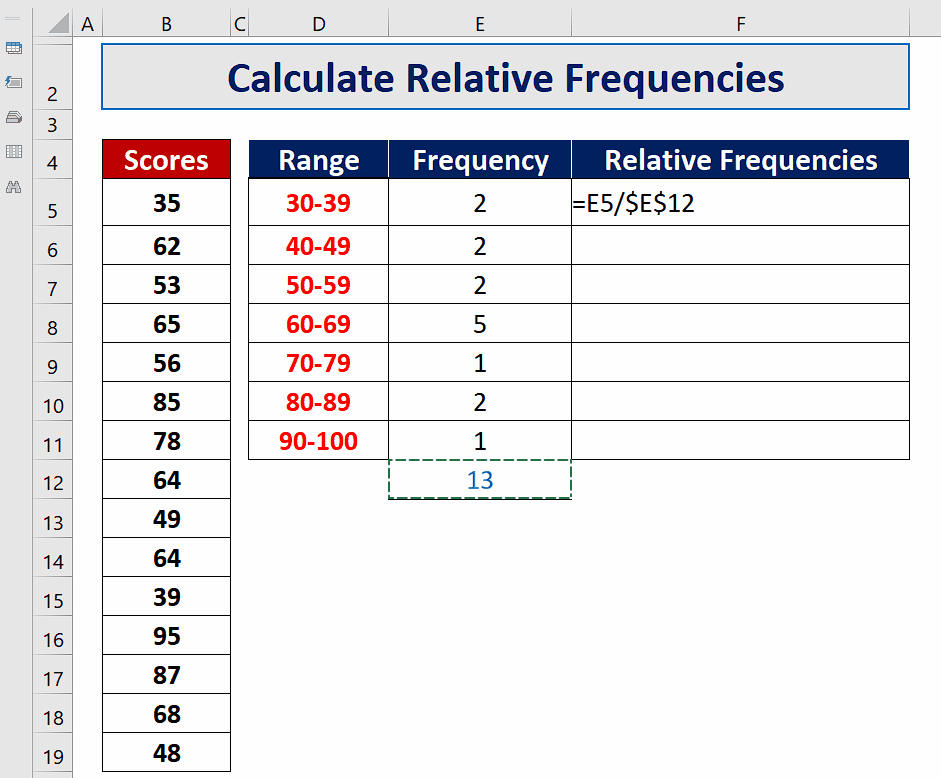# How to calculate relative frequencies in Excel

To understand how to calculate the relative frequencies of a frequency distribution, let’s consider this dataset in the picture above. Our dataset consists of the scores of some students and the data is already available in classes. We have calculated the frequencies of each class and the total of the frequencies is also available.The term frequency tells us how many times an event occurs within a dataset of observations. However, in statistics another term Relative Frequency is used which is a little bit different from simple frequency. In comparison to simple frequency, relative frequency is not the simple count of the event, rather it is the percentage of the count of an event with respect to the total number of events. It is a special kind of frequency and it is represented by percentages, proportions or fractions.

### Step 1 – Write the formula to calculate the relative frequencies– The generic formula to calculate the relative frequencies of each class is as follows;

Relative frequency = f / N

Where,

f = Frequency of a class or range
N = Sum of all frequencies

– However, in our example we have already calculated N i.e. Sum of all frequencies in cell E12. So we’ll divide each class’ frequency with the sum of frequencies and will get the relative frequencies for each class, the actual formula will be;

=E5/\$E\$12

### Step 2 – Implement formula to calculate the relative frequencies– After working out the formula =E5/\$E\$12 we will press enter and the result will be calculated.

– To copy the formula down the column, use the fill handle available at the bottom right corner of the first cell and drag it down the whole column. The usage of absolute reference with E12 cell will keep the calculations intact and we’ll get correct answers in each cell.

– These relative frequencies become more meaningful when they are represented in terms of percentage. Therefore, change the format of the results by selecting all cells and clicking on the percentage (%) icon in the Number group on the Home tab.